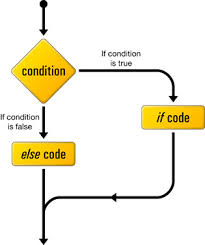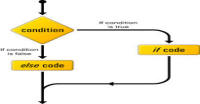# Conditional statements in php

Go back to last tutorial

Show complete series till now

Hello friends,

In this tutorial, we will study about conditional statements (if else statements and switch statements). These statements are used to make decisions with respect to some condition. Lets take an example first:

We have a variable, say \$a, and we want to print 'harish' if \$a is equal to 'harish'. We will write code something like this:

```<?php
\$a = 'harish'; //initialize variable \$a

//check the value of a
if (\$a == 'harish') {
//if \$a is equal to 'harish' then print harish
echo 'harish';
}

?>
```

In this example, you can see that we are using a if statement for checking if value of \$a is equal to 'harish' or not. Focus on the syntax:

The if loop will run if the condition is true or non-zero or non-empty. Here is three words:

1. true: meaning of this word is clear itself. As in example, we have used comparison operator '==' which will check that \$a is equal to 'harish' or not. If yes then it will return true otherwise false. It means condition is true or false.
2. non-zero: We can also experiment by putting 0 and non-zero numbers at the place of condition. In fact, 0 is considered as false and other non-zero letters are considered as true.
3. non-empty: All empty or null variables are considered as false and other variables / values are considered as true. Please not that empty doesn't mean that you leave condition blank. Empty mean null or undefined.

For better understanding, please try all of the three types discussed above.

### if else statement:

This statement is selected when we have to choose one thing out of two things. For example, we want to categorize students of a class on the basis of there marks. If a student gets less than 33 then he will fail otherwise he will pass. Now our script will be like this:

```<?php
\$marks = 23;  // initializing the marks
// change its value for different results

if (\$marks<33) {
echo 'shit! you have failed';
}
else{
echo "you have passed!!!";
}

// above code is equivalent to this code
// remove multiline comment it to verify
/*
if (\$marks<33) {
echo 'shit! you have failed';
}
// >= means less than or equal to
if (\$marks>=33){
echo "you have passed!!!";
}
*/

?>
```

It is clear from example that one of two if or else will run after checking the value of \$marks. else works when condition fails.

### nested statement:

We can use new statement inside another statement. For example if we have to check if student got A+ grade or not then we write script something like this:

```<?php
\$marks = 23;  // initializing the marks
//change its value for different results

if (\$marks<33) {
echo 'shit! you have failed';
}
else{
// we are writing if else inside if else statement

if (\$marks>90) {
echo 'you have passed and got A+ grade';
}
else{
echo 'you have passed but not got A+ grade';
}
}

?>
```

### elseif statements:

If we have more than two choices then we can use elseif statement. It is similar to nested statement. For example, we can write above example something like this:

```<?php
\$marks = 23;  // initializing the marks
//change its value for different results

if (\$marks<33) {
echo 'shit! you have failed';
}
elseif (\$marks>90) {
echo 'you have passed and got A+ grade';
}
else{
echo 'you have passed but not got A+ grade';
}

?>
```

If the first condition fails (marks >= 33) then elseif statement checks its own condition (marks > 90) and if this condition also fails then finally else statement runs. We can write elseif statements any number of times. For example if we have four choices instead of three then we will use elseif statement twice. Try this example yourself and give grade to students.

 Min marks Max marks Grade 0 30 F 31 40 E 41 50 D 51 60 C 61 70 C+ 71 80 B+ 81 90 A 91 100 A+

### switch statement:

If you have done above example yourself then you will found that you have to write conditions again and again. It was a small example what if I give you very large list? Now we will see a new conditional method called switch statements. First look at this code:

```<?php
\$name = 'harish';

switch(\$name){
case 'variable':
echo "it is a variable";
break;
case 'harish':
echo "this is my name";
break;
default:
echo "this is nessary default case";
break; // this statement is not required
}

?>
```

This is a example of switch statement. Lets understand this example:

• We are defining a variable named name
• We are passing that variable to switch statement
• switch behave like electric switch. It takes a value as input. It matches that value to its cases defined inside it. If value matches with any case the program until first time beak statement runs.
• After running first time break statement program comes out from the switch loop.
• Every switch statement must has a default case which runs if the input value does not match with any of the cases.
• We do not require any break statement after default case. We can skip that one.
• In other words, a switch statement is just like group of if else statements. It starts running with a matching (or default) case and ends with break or '}'.

Advantage of switch statement is that it reduces the length of our code. Now return to our student's grading example and try to solve that with switch statement. We will get this code.

```?php
\$marks = 0;  // initializing the marks
//change its value for different results

// we are rounding marks to one digit
// keeping same grade marks together
\$m = (\$marks - 1)/10;

// intval changes floating value to integer value
// for example 3.2 => 3  and 3.8 => 3
switch (intval(\$m)) {
// case continue untill break
case 0:
case 1:
case 2:
echo "F";
break;
// after break statement program leaves the switch loop
case 3:
echo "D";
break;
case 4:
echo "C";
break;
case 5:
echo "C+";
break;
case 6:
echo "B";
break;
case 7:
echo "B+";
break;
case 8:
echo "A";
break;
case 9:
echo "A+";
break;
default:
// default case if none of the case satisfied
echo "wrong input for marks";
break;
}

?>
```

From the program, it is clear that you do not have to write much code just like before. Lets know what is going on in the code:

• First we are subtracting one from the marks to make first digit of each grade ( except F) same. For example, 50 becomes 49 and 100 becomes 99.
• Now marks are divided by 10 to make marks in a range of -0.1 to 9.9. For example, 00 becomes 0.1 and 67 becomes 6.7
• intval is PHP inbuilt function which returns the integer part of the number. For example, it return 3 for 3.4, 3.5 and 3.8. In short, switch is taking integer part as an input.
• After it we are matching that integer with cases. As I told before the case will be continued until the break statement. So, case 0, 1 and 2 are pointing to same steps, i.e. prints 'F' because there is no break statement between them.

### Exercise:

• Try to modify these examples.
• Think more problems and try to solve them if you find any difficulty then ask in comments.

In next tutorial we will study about loops in PHP. If you like it then please share it with your friend otherwise give your valuable feedback to improve this as well as future tutorial to help other people.

Don't forget to Subscribe for latest feeds.

next tutorialHarish, a fullstack developer at www.lyflink.com with five year experience in full stack web and mobile development, spends most of his time on coding, reading, analysing and curiously following businesses environments. He is a non-graduate alumni from IIT Roorkee, Computer Science and frequently writes on both technical and non-technical topics.

### Related ArticlesPHP stands for PHP: Hypertext Preprocessor. PHP is known as a server side scripting and interpreter language for web ...
##### Introduction to PHPIn this tutorial, we will study about conditional statements (if else statements and switch statements). These statem...
##### Conditional statements in phpIn this tutorial, we will study about strings and operations upon strings. Due to long length of this section, I have...

### Complete Python Bootcamp: Go from zero to hero in Python 3### The Complete Web Developer Course - Build 25 Websites